Xue, B., and Hu, Y. (2013). "Analysis of the microstructure and mechanical properties of laminated veneer lumber," BioRes. 8(2), 2681-2695.

#### Abstract

In this paper, four different nondestructive testing (NDT) methods and static bending tests were done on poplar (Populus ussuriensis Kom.) and birch (Betula platyphylla Suk.) Laminated Veneer Lumber (LVL). The effects of compression ratio on the modulus of elasticity (MOE) and modulus of rupture (MOR) of LVL with vertical load and parallel load were investigated. There were four compression ratios: 8.1%, 18.3%, 26.5%, and 33.1%. The microscopic structure of LVL was analyzed with a scanning electron microscope (SEM). Results showed a strong correlation between each dynamic Young’s modulus and the static MORof LVL; the MOE and MOR of LVL changed with the increase of compression ratio. MOE and MOR were greatly increased when the compression ratio increased from 18.3% to 26.5%, and the microstructure of LVL changed greatly between different compression ratios by birch and poplar species.

Analysis of the Microstructure and Mechanical Properties of Laminated Veneer Lumber

Bing Xue,a,b and Yingcheng Hu a,*

In this paper, four different nondestructive testing (NDT) methods and static bending tests were done on poplar (Populus ussuriensis Kom.) and birch (Betula platyphylla Suk.) Laminated Veneer Lumber (LVL). The effects of compression ratio on the modulus of elasticity (MOE) and modulus of rupture (MOR) of LVL with vertical load and parallel load were investigated. There were four compression ratios: 8.1%, 18.3%, 26.5%, and 33.1%. The microscopic structure of LVL was analyzed with a scanning electron microscope (SEM). Results showed a strong correlation between each dynamic Young’s modulus and the static MOR of LVL; the MOE and MOR of LVL changed with the increase of compression ratio. MOE and MOR were greatly increased when the compression ratio increased from 18.3% to 26.5%, and the microstructure of LVL changed greatly between different compression ratios by birch and poplar species.

Keywords: Laminated veneer lumber (LVL); Compression ratio; Nondestructive testing (NDT); Mechanical properties; Microstructure; Birch; Poplar

Contact information: a: Key Laboratory of Bio-based Material Science and Technology of Ministry of Education of China, College of Material Science and Engineering, Northeast Forestry University, Harbin, 150040, China; b: Heilongjiang Institute of Science and Technology, Harbin, 150027, China;

* Corresponding author: yingchenghu@163.com

INTRODUCTION

In view of the increasing awareness of society concerning the natural environment, the acceptance of wooden building materials in the form of solid wood and wood-based composites has increased substantially during the past few decades. The main advantages of these materials are availability, renewability, lower processing costs, and simplicity of dismounting and disposal at the end of their service life. Researchers are showing increased interest in the benefits of composite technology for wood-based materials for structural and non-structural usage (Fridley 2002). One of the objectives of composite technology is to produce a product with acceptable performance characteristics using low quality raw materials, combining beneficial aspects of each constituent. New composites are produced with the aim to reduce costs and to improve performance (Schuler and Adair 2003).

Laminated Veneer Lumber (LVL) has been developed as an alternative to solid wood. Veneers obtained from medium- or small-diameter logs are converted into glued parallel laminates or Laminated Veneer Lumber (LVL) which has all the properties of thick wooden planks and it is a useful product for structural purposes. Detailed infor-mation on production techniques, technological properties, advantages, and disadvan-tages of these types of panel products can be found in the literature (Kamala et al. 1999; Semra et al. 2007).

The field of Nondestructive Testing (NDT) is a very broad, interdisciplinary field that plays a critical role in ensuring that structural components and systems perform their function in a reliable and cost-effective fashion. The utilization of nondestructive testing (NDT) in the evaluation of materials property and performance makes it possible to control the product quality, to monitor equipment operation, to evaluate structure integrity, and to predict residual life of components. NDT has the advantage of not only being prompt, convenient, and time-saving, but also giving great financial benefits (Sinclair 1989; Chen et al. 1999). NDT was investigated on poplar lumber and fiber-reinforced plastic (FRP) reinforced fast-growing poplar glulam. Results indicated that the NDT could predict static properties of both wood products. The dynamic Young’s modulus values of FRP reinforced the idea that poplar glulam obtained by the dynamic test were generally higher than those by static testing. Reliability of timber structure design based on predicted modulus of rupture (MOR) poplar lumber was less than that on measured MOR (Cheng and Hu 2011a, b).

The physical and mechanical properties of LVL are significant parameters in the adhibition of LVL, and these properties have been widely studied by researchers working in the field of wood science and technology. But there has been less research aimed at obtaining physical and mechanical properties of LVL through the use of NDT methods or by analysis of the microscopic structure with a scanning electron microscope (SEM) (Dobmann et al. 1997; Hu and Afzal 2006).

There are many factors that affect the mechanical properties of LVL, such as the compression ratio, the wood species, veneer drying and aging, size and the density of LVL, and so on (Semra et al. 2007; Shukla and Kamdem 2008; Fonselius 1997). The purpose of this study was to analyze the effect of compression ratio and wood species on the mechanical properties of LVL. A further goal was to evaluate the feasibility of using the composite material mechanics analysis method with the NDT testing of LVL. Three analytical methods were used to analyze the mechanical properties of LVL: composite material mechanics, the NDT method, and static testing. In order to analyze the variety of microscopic structures between different compression ratios, the microscopic structure of the LVL was analyzed with SEM.

EXPERIMENTAL

Wood Materials

The rotary-cut veneers were made from poplar (Populus ussuriensis Kom.) and birch (Betula platyphylla Suk.). The poplar and birch trees were harvested from Inner Mongolia. Round logs obtained from the trees were cut into stocks in rough sizes by taking into consideration final layer dimensions of 600 mm × 500 mm × 3.4 mm (length×width×thickness). A special emphasis was placed on the selection of the wood material. Accordingly, non-deficient, proper, knotless, and normally grown (without zone line, reaction wood, decay, insect, and fungal damages) wood materials were selected, making sure that the growth rings were perpendicular to the surface. The stocks were dried in a drying kiln until a moisture content of 7±1% was reached. These stocks were later used to make 25 mm-thick eight-ply, nine-ply, ten-ply, and eleven-ply LVL in the laboratory.

Preparation of LVL Panels

The LVL panels were bonded with a commercial phenol-formaldehyde (PF) resin. The glue was spread at a rate of 150 g/m2 onto a single surface of each layer. Glue was spread uniformly on the veneers by manually hand brushing. The glued layers were brought together immediately, one on top of the other, and were kept this way for 30 min before being hot-pressed in a pressing machine for a duration of 40 min under a pressing temperature of 160 ºC and pressure of 1.5 MPa. The target thickness of LVL panels was 25 mm. There were four compression ratios for LVL samples having different numbers of plies: 8.1% for eight-ply samples, 18.3% for nine-ply samples, 26.5% for ten-ply samples, and 33.1% for eleven-ply samples, respectively.

Preparation of Test Samples

Test samples with dimensions of 575 mm × 90 mm × 25 mm and 575 mm × 25 mm × 25 mm were obtained from the LVL panels. The test samples dimension of 575 mm × 90 mm × 25 mm were used in experiments vertical to the glue-line direction load, and the test samples dimension of 575 mm × 25 mm × 25 mm were used in experiments parallel to the glue-line direction load. All test samples were put in an acclimatization chamber, in which the temperature was 20±2 ºC, and the relative humidity was 65±5%, until the weights of the samples remained constant, for the purpose of homogenization of moisture by volume before the experiments. All specimens were tested for the dynamic Young’s modulus by NDT testing first, and then MOE and MOR by static testing.

Nondestructive Testing

NDT and static tests of vertical load and parallel load research were conducted to evaluate the mechanical properties of the LVL. The values of the dynamic Young’s modulus were obtained by using three different NDT methods, i.e. the flexural vibration method (out-plane and in-plane), the longitudinal vibration method, and the longitudinal transmission test (Hu 2004; Hu et al. 2005 a, b).

The experiment was first carried out with the flexural vibration method as shown in Figs. 1 and 2. In the test, specimens in the freely vibrating free-free beam test were supported by two planks. The supporting positions of the planks were 0.224 Ls (length of specimen) from both ends. This position corresponds to the nodal points for the fundamental mode of this vibration system. The vibrating frequency was detected by a high-sensitivity microphone connected to a FFT analyzer. The resonant frequencies of the 1st, 2nd, 3rd, and 4th modes were obtained by giving a blow to an edge of the beam and recording the results with the FFT analyzer. The dynamic MOE was obtained from Timoshenko-Goens-Hearmon (TGH) flexural vibration method including the influence of shear and rotatory inertia (Hu 2004).

The experiment was then carried out with the longitudinal vibration method, as shown in Fig. 3. In the test, the specimen was held lightly by the fingers at the center of the specimen while they were tapped by a small hammer at the end of the specimen. The tap tone was detected by a microphone at the other end of the beam. The resonance frequencies of the tap tone were identified by a FFT analyzer. The dynamic MOE was calculated by Eq. 1 (Hu 2004),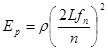, n=1, 2, 3…… (1)

where Ep is dynamic MOE of specimen, L is the length of the specimen, fn is the resonance frequency, and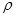is the density of the specimen.

The experiment was last carried out with the longitudinal transmission method as shown in Fig. 4. In the test, the sound transmission time propagating through the specimen was measured with a fast Fourier transform (FFT) analyzer. The sound velocity and dynamic MOE were calculated based on Eqs. 2 and 3 (Hu 2004),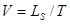(2)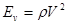(3)

where V is sound velocity, L is length of the specimen, T is transmission time, Ev is dynamic MOE, andis the density of the specimen.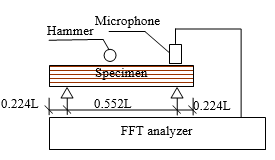Fig. 1. Out-plane flexural vibration test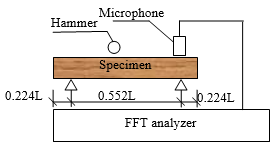Fig. 2. In-plane flexural vibration test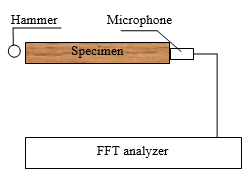Fig. 3. Longitudinal vibration test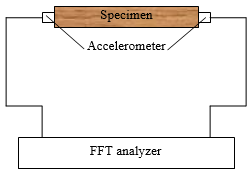Fig. 4. Longitudinal transmission test

Microscopic Structure Testing

The 1 cm3 blocks were pre-prepared from LVL specimens. The endgrain was given a cursory polish with a razor blade so that the tangential direction could be located under a dissecting microscope. A second split was made along the tangential surface so that a sample with a polish flat top and bottom was created. Then the samples were observed under electron microscopy after applying a thin layer of gold by sputter-coating. SEM was used to investigate the morphology of samples by using the FEI Model Quanta 200 (FEI Company, USA), and the samples were observed using an applied tension of 12.5 kV. The microscopic structure photos were magnified, the percentage of cell wall was measured with area method and weight method by TDY-5.2 color image computer analysis software packages.

Static Testing

The static bending tests were conducted on the specimens in accordance with the Japanese Agricultural Standard (JAS: SE-11 No. 237: 2003) of Structural Laminated Veneer Lumber. The static MOR in bending and modulus of elasticity (MOE) in bending was calculated based on Eqs. 4 and 5,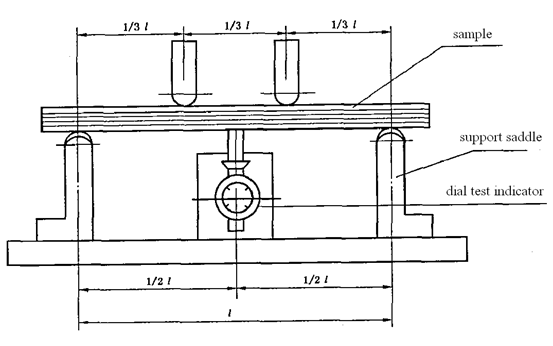Fig. 5. Vertical load static test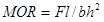(4)

where MOR is the modulus of rupture (MPa), is the maximum load (N), l is the span in bending between the testing machine grips (mm), b is the cross sectional width in the bending test (mm), and his the cross sectional thickness in the bending test (mm).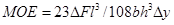(5)

In Eq. 5, MOE is the modulus of elasticity (GPa), ΔF is the increment of load on the regression line with a correlation coefficient of 0.99 or better (N), Δy is the increment of deformation corresponding to F2 – F(N), and lb, and, h are the same as in Eq. 4.

Material Mechanics Analysis

According to previous research (Xue and Hu 2012; Gibson 2011), the following expressions were found by introducing the theoretics of elasticity mechanics,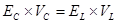(6)

where Ec is the static test longitudinal MOE of uncompressed clear wood sample, Vc is the volume of uncompressed clear wood sample, EL is the static test longitudinal MOE of LVL sample, and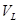is the volume of LVL sample.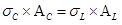(7)

In Eq. 7, c is the static test longitudinal ultimate pull stress of uncompressed clear wood sample, Ac is the cross-sectional area of uncompressed clear wood sample, L is the static test longitudinal ultimate pull stress of LVL sample, and AL is the cross-sectional area of LVL sample.

RESULTS AND DISCUSSION

In order to show clearer correlations of every dynamic Young’s Modulus of each LVL specimen, the test results of poplar LVL specimen were chosen. The average values of the dynamic Young’s modulus and MOR are shown in Fig. 6. As shown, the Eof poplar LVL, dynamic Young’s modulus by longitudinal transmission method, was the largest value of all dynamic Young’s modulus values. There was little difference between E1E2, and Ep, where the E1 is dynamic Young’s modulus by out-of-plane flexural vibration method, E2 is dynamic Young’s modulus by in-plane flexural vibration method, and Ep is dynamic Young’s modulus by longitudinal vibration method, respectively. The trend curve of each NDT test result and MOR was very similar.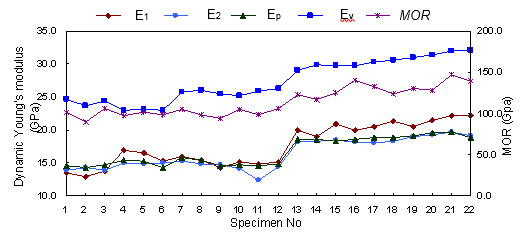Fig. 6. The values of dynamic Young’s modulus of different NDT testing methods and MOR of each poplar LVL specimen with vertical load

The regression curves between various dynamic Young’s modulus and the MOR of all poplar LVL specimens are shown in Fig. 7. From the regression analysis between various dynamic Young’s modulus and MOR, the linear regression formulas were obtained and shown in Tables 1 and 2. Each correlation coefficient between dynamic Young’s modulus and MOR was much greater than R20, 0.01= 0.537. The correlation coefficient of poplar LVL was larger than each of the NDT methods with vertical load, and the correlation coefficient of birch was larger with parallel load. Thus, there was a strong correlation between each dynamic Young’s modulus and the static MOR; the four different NDT methods can be applied to test the MOR of birch and poplar LVL entirely.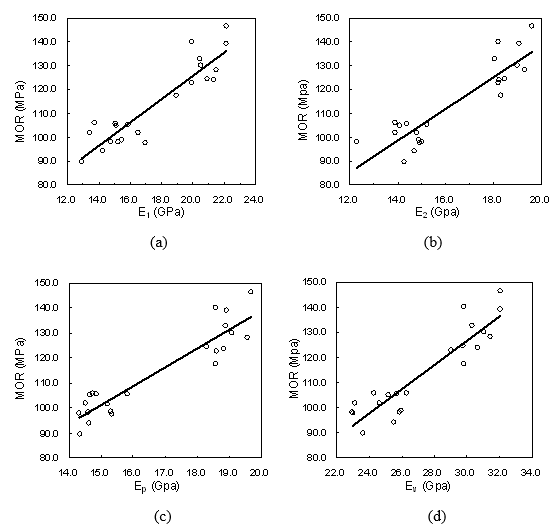Fig. 7. Regression curve between various dynamic Young’s modulus and MOR of poplar LVL with vertical load

Table 1. Linear Regression Formula and Correlation Coefficients of Vertical Load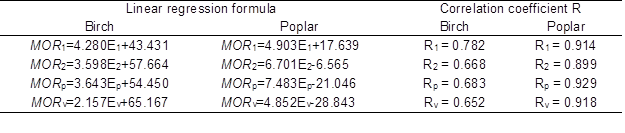R1 is correlation coefficient of out-of-plane flexural vibration method, R2 is correlation coefficient of in-plane flexural vibration method, Ris correlation coefficient of longitudinal vibration method, and Rv is correlation coefficient of longitudinal transmission method

Table 2. Linear Regression Formula and Correlation Coefficients of Parallel Load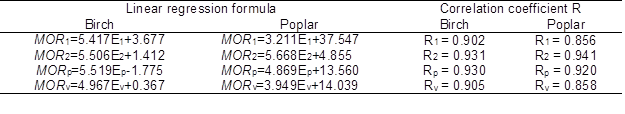The value of R1 was greater than that of R2 for both birch and poplar LVLs in the case of vertical load, whereas the relationship was the reverse in the case of birch and poplar LVLs with parallel load. This was because the direction of striking in the out-of-plane or in-plane flexural vibration method was in accordance with the loading direction of static bending test.

The effects of compression ratio on static testing MOE and MOR of LVL by vertical load and parallel load are described by Figs. 8 and 9. The MOE and MOR of birch LVL were larger than poplar LVL (Figs 8 and 9). The mechanical properties of birch LVL was more than poplar LVL. The amplitude of variation of birch LVL MOE was larger than poplar LVL; the effects of compression ratio on mechanical properties of birch LVL was less than poplar LVL, and the mechanical properties of birch LVL was relatively stable.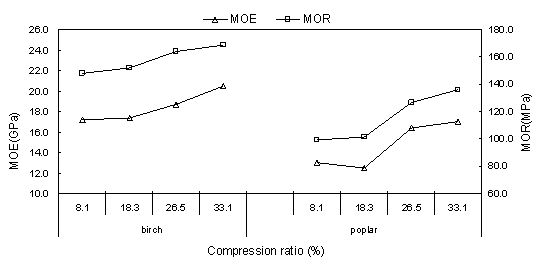Fig. 8. Effects of compression ratio on static testing MOE and MOR of LVL with vertical load

The MOE and MOR of birch LVL increased with an increase of compression ratio with both load methods. Moreover, most MOE and MOR of poplar LVL increased with an increase of compression ratio with both load methods. While the anomalous data included that among poplar LVL samples, the MOE of compression ratio 18.3% was less than 8.1% with two load methods, and the MOE and MORof compression ratio 33.1% was less than 26.5% with parallel load. So there were significant effects of the compression ratio on MOE or MOR. But MOE and MOR of poplar LVL were not in accordance with this rule entirely, possibly because poplar is a fast-growing wood species and its cell wall structure is unstable. In order to find the reason, the microscopic structure was analyzed in this study.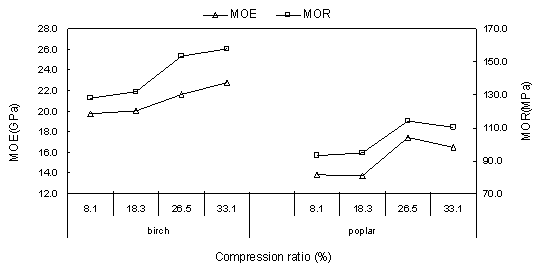Fig. 9. Effects of compression ratio on static testing MOE and MOR of LVL with parallel load

The EC and c theoretical average results and coefficient of variation (COV) of the different compression ratio LVL were obtained by static test results, using Eqs. 6 and 7, and they are presented in Tables 3 and 4.

Table 3. Theoretical Results of Vertical Load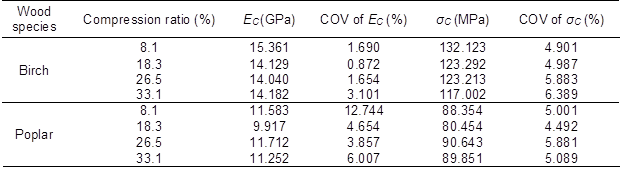Table 4. Test and Theoretical Results of Parallel Load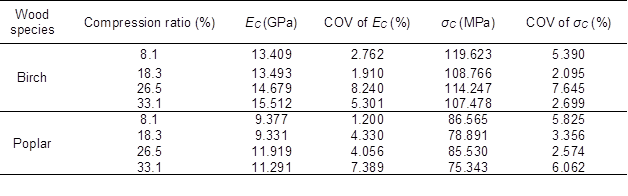According to Tables 3 and 4, the average of birch and poplar vertical load EC was 14.418 and 11.116 GPa, respectively, and the parallel load EC was 14.273 and 10.479 Gpa, respectively. The average of birch and poplar vertical load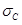was 123.901 and 87.326 MPa, respectively, and the parallel loadwas 112.529 and 81.582 MPa, respectively. These data are similar to the congeneric data in some interrelated references (Bodig and Jayne 1982; Liu 2004; Long 2005). Most COVs of EC andwere under 10%, and the results were very steady. Thus, Eqs. 6 and 7 were judged to be workable, on a rudimentary level, but the half Eandof compression ratio 33.1% was less than 26.5% with two load methods.

As mentioned above, the MOE and MOR of the larger compression ratio LVL was less than that of the smaller compression ratio LVL. In order to explain this phenomenon, the microscopic structure of LVL was observed with SEM and is described by Figs. 10 and 11.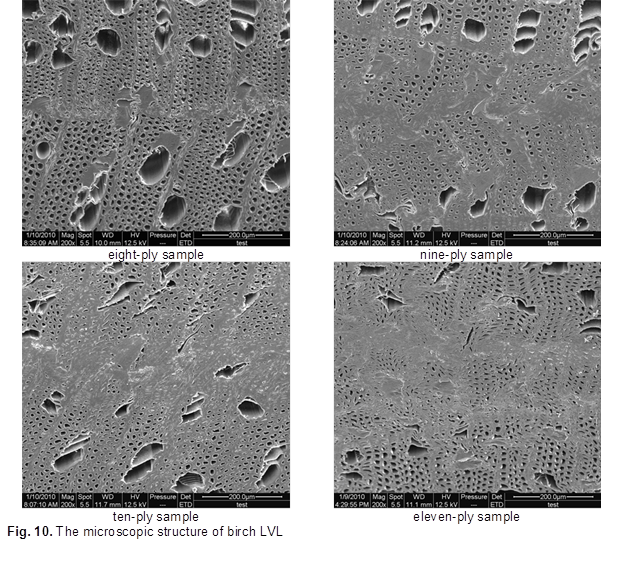According to Fig. 10, the dimensions of vessels decreased as the compression ratio increased, and the lumina near the glue line were compressed slightly. There was no compression failure in the birch sample subject to different compression ratios, so the MOE and MOR increased with the increase of compression ratio.